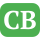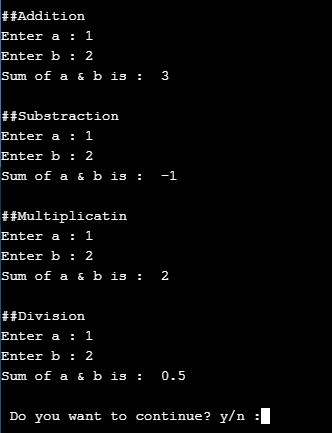CppBuzz.com

# Python program on Addition, Substration, Multiplication and Division

This program take input a & b from user and prints their addition, substration, multiplication, division. Program is compiled on Python 3 Version

``````choice = 'y'

while choice=='y':
a = int(input('Enter a : '))
b = int(input('Enter b : '))
c = a + b;
print('Sum of a & b is : ', c);
print('\n##Substraction')
a = int(input('Enter a : '))
b = int(input('Enter b : '))
c = a - b;
print('Sum of a & b is : ', c);
print('\n##Multiplicatin')
a = int(input('Enter a : '))
b = int(input('Enter b : '))
c = a * b;
print('Sum of a & b is : ', c);
print('\n##Division')
a = int(input('Enter a : '))
b = int(input('Enter b : '))
c = a / b;
print('Sum of a & b is : ', c);

choice = input('\n Do you want to continue? y/n :')

```
```